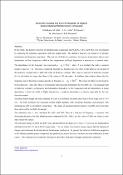## Dielectric Response and Electric Properties of Organic Semiconducting Phthalocyanine Compounds2011-05-04
##### Authors
AbdelKarim M. Saleh
S. M. Hribat
R. Kitaneh
M. Abu-Samreh
M. Abu-Samreh
##### Abstract
<p>In this study, the dielectric function of phthalocyanine compounds (ZnPc, H2Pc, CuPc, and FePc) were investigatedby analyzing the measured capacitance and loss tangent data. The dielectric function was found to be stronglytemperature and frequency dependent. The real part of dielectric constant ε1 increases at temperatures above roomtemperature and low frequencies, while at low temperatures and high frequencies it saturates to a constant value.The dependence on the frequency was expressed as: s1 A, where A is a constant, the index s assumesnegative values (s &lt; 0). The type of electrode material was found to have no effect on the behavior of real part ofthe dielectric constant but it is affect the value of dielectric constant. The values of real part of dielectric constantfor Au electrodes are larger than those of the values of Al electrodes. In addition, data analysis shows that theimaginary part of dielectric constant depends on frequency as m2 B. The values of index m were found tobe less than zero. Also, the values of m decreases with increasing temperature for Au-ZnPc-Au. The imaginary partof dielectric constant ε2 is frequency and temperature dependent. At low frequencies and all temperatures, a strongdependence is observed, while at higher frequencies, a moderate dependence is obvious especially for the Auelectrodesample.Maximum barrier height has been estimated for ZnPc with different electrodes and found to be in range of (0.17 to 4eV). For both electrodes the maximum barrier height increases with increasing frequency and decreases withtemperatures until to zero Kelvin temperature. The values of maximum barrier height of Al-ZnPc-Al are less thanthose observed for Au-ZnPc-Au sample.The relaxation time, τ, was calculated for ZnPc and FePc films as a function of frequency. τ increases withdecreasing frequency for both phthalocyanine compounds (ZnPc, FePc), but the values of ZnPc are closer to eachother than FePc values.The activation energy of ZnPc and FePc were calculated from the slopes of τ versus 1/T curves at low temperaturesand found to be 0.01 eV and 0.04 eV respectively. The low values of activation energy suggest that the hopping ofcharge carriers between localized states is the dominant mechanism. In general, the behavior of dielectric propertiesof the different phthalocyanine compounds are qualitatively almost the same, but there are some differences in theirvalues. This variation may be related to the type of electrodes, thickness of sample, and growth conditions.</p>
<p>In this study, the dielectric function of phthalocyanine compounds (ZnPc, H2Pc, CuPc, and FePc) were investigatedby analyzing the measured capacitance and loss tangent data. The dielectric function was found to be stronglytemperature and frequency dependent. The real part of dielectric constant ε1 increases at temperatures above roomtemperature and low frequencies, while at low temperatures and high frequencies it saturates to a constant value.The dependence on the frequency was expressed as: s1 A, where A is a constant, the index s assumesnegative values (s &lt; 0). The type of electrode material was found to have no effect on the behavior of real part ofthe dielectric constant but it is affect the value of dielectric constant. The values of real part of dielectric constantfor Au electrodes are larger than those of the values of Al electrodes. In addition, data analysis shows that theimaginary part of dielectric constant depends on frequency as m2 B. The values of index m were found tobe less than zero. Also, the values of m decreases with increasing temperature for Au-ZnPc-Au. The imaginary partof dielectric constant ε2 is frequency and temperature dependent. At low frequencies and all temperatures, a strongdependence is observed, while at higher frequencies, a moderate dependence is obvious especially for the Auelectrodesample.Maximum barrier height has been estimated for ZnPc with different electrodes and found to be in range of (0.17 to 4eV). For both electrodes the maximum barrier height increases with increasing frequency and decreases withtemperatures until to zero Kelvin temperature. The values of maximum barrier height of Al-ZnPc-Al are less thanthose observed for Au-ZnPc-Au sample.The relaxation time, τ, was calculated for ZnPc and FePc films as a function of frequency. τ increases withdecreasing frequency for both phthalocyanine compounds (ZnPc, FePc), but the values of ZnPc are closer to eachother than FePc values.The activation energy of ZnPc and FePc were calculated from the slopes of τ versus 1/T curves at low temperaturesand found to be 0.01 eV and 0.04 eV respectively. The low values of activation energy suggest that the hopping ofcharge carriers between localized states is the dominant mechanism. In general, the behavior of dielectric propertiesof the different phthalocyanine compounds are qualitatively almost the same, but there are some differences in theirvalues. This variation may be related to the type of electrodes, thickness of sample, and growth conditions.</p>